Mathematics
Easy

Question

# Identify the correct graph for the equation. y =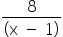–2

##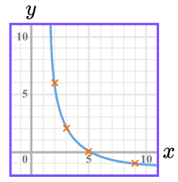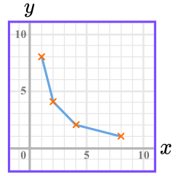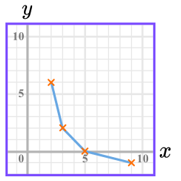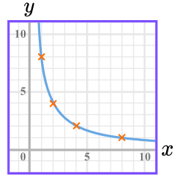Hint:

## The correct answer is:### Seeing the options, let’s check the point (5, 0). If x = 5, y =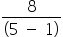– 2 = 0 So, (5, 0) passes through the graph. That means it can be either graph A or graph C. It is graph A as it is a reciprocal function and it has the shape of a hyperbola.Hence, the correct option is A.

We can substitute the points lying on the graph to determine which is the graph of the given equation.

### Related Questions to study#### With Turito Foundation.#### Get an Expert Advice From Turito.